# pbrt-v2 加速結構 BVH-contract 改寫

### Description of implementation approach and comments

1. 利用原生方法建立 full binary tree 的 BVH (利用各種分割策略完成)
2. 進行坍倒 (flatten)，將二元樹不連續的記憶體分布調整成線性分布，加速遍歷走訪的內存快取效率。
3. 靜態調整成 generic tree 版本，藉由啟發式收縮 (Contract)，利用節點與其子節點的 Bounding Box 表面積比例，評估浪費的走訪節點。
4. 動態調整部分，採用隨機取樣，根據取樣 ray，取樣走訪次數，將比較容易打到的節點盡可能收縮到接近根節點。

\begin{align} \delta(N) &= n_{N.\text{child}} \; C_B - (\alpha_N (1+n_{N.\text{child}}) +(1 - \alpha_N)) \; C_B \\ & = ((1 - \alpha_N) \; n_{N.\text{child}} - 1) \; C_B \end{align}

$\alpha(N) > 1 - \frac{1}{n_{N.\text{child}}}$

### Generic Tree 設計與走訪測試

#### 節點大小對走訪效能影響

sizeof(LinearTreeNode) bytes \ Traversal Recursive Loop
32 6.049s 5.628s
44 6.651s 6.817s
60 7.460s 6.888s
92 9.361s 9.271s
156 16.844s 16.694s
220 25.294s 27.031s
284 28.181s 30.900s
540 28.560s 33.707s

### 實驗結果

sences \ BVH policy Native Contract(loop) Contract(recursive) Node Reduce
sibenik.pbrt 7.000s 10.502s 9.411s 99576 / 131457
yeahright.pbrt 12.297s 14.638s 14.210s 288707 / 376317
sponza-fog.pbrt 16m36.037s 21m09.960s 20m12.012s 91412 / 121155

### Reference paper

Ray Specialized Contraction on Bounding Volume Hierarchies, Yan Gu Yong He Guy E. Blelloch

Read More +

# pbrt-v2 加速結構 Environment Lights 改寫

### Median Cut Algorithm

#### 在 Median Cut Algorithm 不同數量 equal-energy region 在 256 samples 速度

##### Median Cut Algorithm equal-energy region

1. 將入射光場影像 (light probe image) 切成好幾個矩形區域，每一個區域將取用一個點光源代替。將入射光場影像轉換成灰階亮度 $Y$，如論文中所提及的方案 $Y = 0.2125 R + 0.7154 G + 0.0721 B$ 這類型的轉換。
2. 對於每一個區域將會根據最長維度切割成兩個子區域。切割成兩個子區域的亮度總和越接近越好。
3. 若切割區域數量不到目標的數量，則重複步驟 2。
4. 最後將每一個區域算出代表的點光源，並加總區域內的亮度和，隨後取樣根據亮度和作為取樣根據 (在 Spectrum MedianCutEnvironmentLight::Sample_L(const Point&, float, const LightSample&, float, Vector&, float*, VisibilityTester*) 中使用)，用每一個區域內部的 pixel 位置和亮度計算重心作為代表的點光源。

Median Cut Algorithm 需要 $\mathcal{O}(HW)$ 時間 $\mathcal{O}(HW)$ 空間來預處理亮度資訊。若要切割成 $N$ 個區域，需要 $\mathcal{O}(\log N)$ 次迭代，每一次迭代增加兩倍區域數量。將一個區域切割時，針對維度最長的那一軸二分搜尋，二分搜尋計算其中一個區域的亮度和是否是整個區域亮度的一半，由於預處理完成的表格，可以在 $\mathcal{O}(1)$ 時間內計算任意平行兩軸的矩形內部元素總和。

### Centroid Formula 1

$$X_c = \frac{\sum^{}_{(x, y) \in \mathit{region}} L_{(x, y)} \; x}{\sum^{}_{(x, y) \in \mathit{region}} L_{(x, y)}} \\ Y_c = \frac{\sum^{}_{(x, y) \in \mathit{region}} L_{(x, y)} \; y}{\sum^{}_{(x, y) \in \mathit{region}} L_{(x, y)}}$$

### Centroid Formula 1

$$X_c = \frac{\sum^{}_{(x, y) \in \mathit{region}} L^2_{(x, y)} \; x}{\sum^{}_{(x, y) \in \mathit{region}} L^2_{(x, y)}} \\ Y_c = \frac{\sum^{}_{(x, y) \in \mathit{region}} L^2_{(x, y)} \; y}{\sum^{}_{(x, y) \in \mathit{region}} L^2_{(x, y)}}$$

Read More +

# pbrt-v2 加速結構 Realistic Camera 改寫

### Ray Sphere Intersection

• $\overrightarrow{OC} + \overrightarrow{I} \times t = \overrightarrow{OP}$
• $|\overrightarrow{OP}| = \text{radius}$
• $|\overrightarrow{OC} + \overrightarrow{I} \times t| = |\overrightarrow{OP}|$
• $|\overrightarrow{I}|^2 t^2 + 2 \; (\overrightarrow{OC} \cdot \overrightarrow{I}) \; t + |\overrightarrow{OC}|^2 - \text{radius}^2 = 0$### Snell’s Law

#### Whitted’s Method

$\sqrt{}$ $/$ $\times$ $+$ compute
1 $n = \eta_1 / \eta_2$
3 3 2 $I' = I / (-I \cdot N)$
3 $J = I' + N$
1 1 8 5 $\alpha = 1 / \sqrt{n^2(I' \cdot I') - (J \cdot J)}$
3 3 $T' = \alpha J - N$
1 3 3 2 $T' = T' / \| T' \|$
2 8 17 15 TOTAL

#### Heckbert’s Method

$\sqrt{}$ $/$ $\times$ $+$ compute
1 $\eta = \eta_1 / \eta_2$
3 2 $c_1 = - I \cdot N$
1 3 2 $c_2 = \sqrt{1 - \eta^2(1 - c_1^2)}$
7 4 $T = \eta I + (\eta c_1 - c_2) N$
1 1 13 8 TOTAL

#### Other Method

$\sqrt{}$ $/$ $\times$ $+$ compute
1 $n = \eta_2 / \eta_1$
3 2 $c_1 = - I \cdot N$
1 2 3 $\beta = c_1 \sqrt{n^2 - 1 + c_1^2}$
3 3 3 $T = (I + \beta N ) / n$
1 4 8 8 TOTAL

### RasterToCamera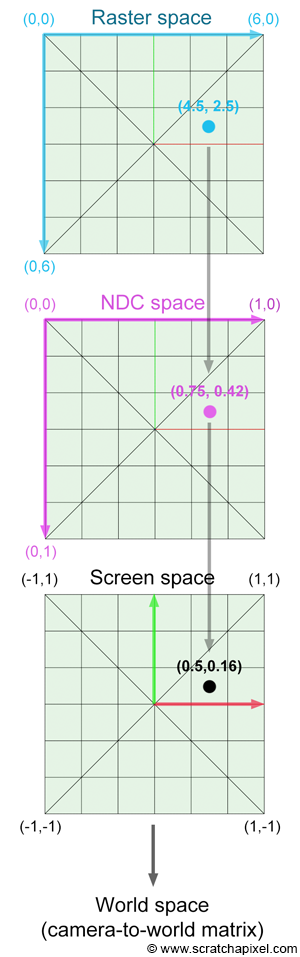• Raster-To-NDC 轉換矩陣 $A=\text{Scale}(scale, scale, 1)$，進行縮放。
• NDC-To-Camera 轉換矩陣 $B=\text{Translate}(Vector(0, 0, filedistance)) \times \text{Translate}(Vector(X, -Y, 0))$，進行位移。
• 最後得到 RasterToCamera 轉換矩陣 $C= B \times A \times \text{Scale}(-1, 1, 1)$，最後將 x 軸顛倒以符合成像問題。

### Ray Weight

If the exit pupil subtends a small solid angle from $x'$, $\theta'$ can be assumed to be constant and equal the angle between $x'$ and the center of the disk. This allows us to simplify
$E(x') = \int_{x'' \in D} L(x'', x') \frac{\cos \theta' \cos \theta''}{\| x'' - x'\|} dA''$<span>$to:$</span><!-- Has MathJax -->$E(x’) = L \frac{A}{Z^2} \cos^4 \theta’$
where $Z$ is the axial distance from the film plane to the dist and $A$ is the area of the disk.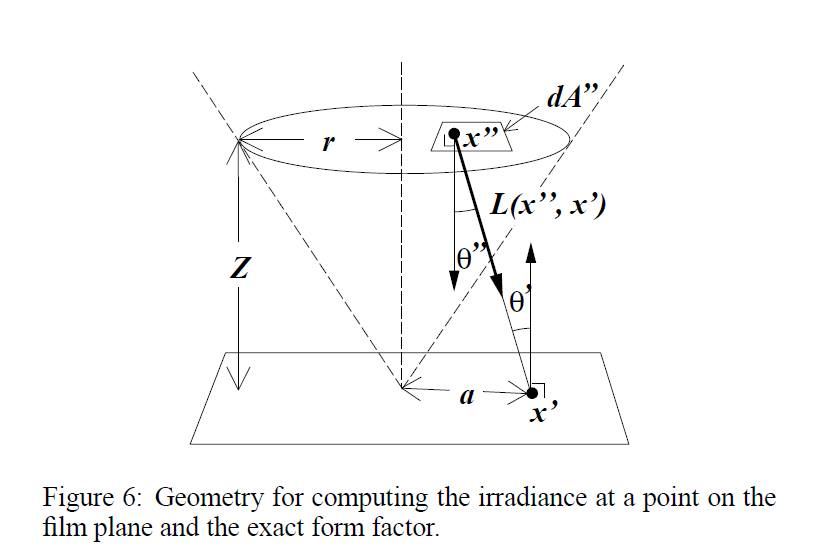## Final Images Rendered Compare 4 samples

#### Gauss Lens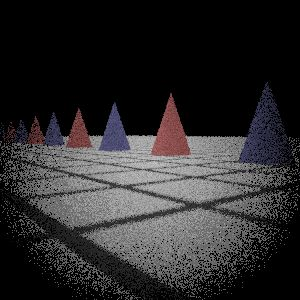My Implementation (Sampling 1)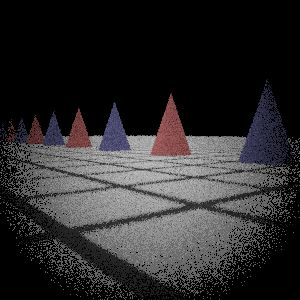My Implementation (Sampling 2)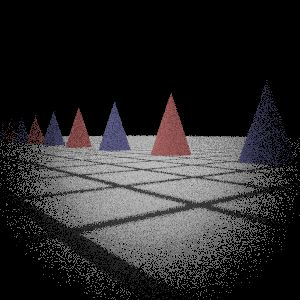My Implementation (Sampling 3)

#### Fisheye Lens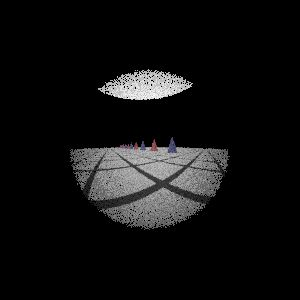My Implementation (Sampling 1)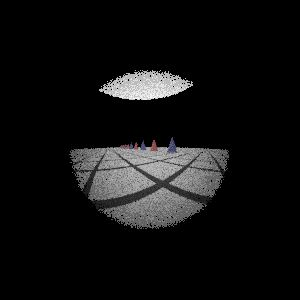My Implementation (Sampling 2)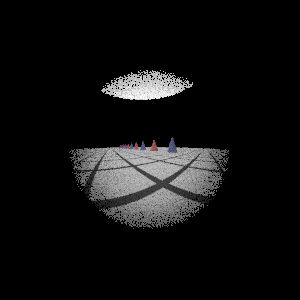My Implementation (Sampling 3)

#### Telephoto Lens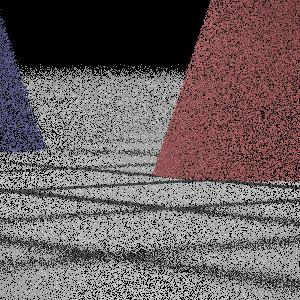My Implementation (Sampling 1)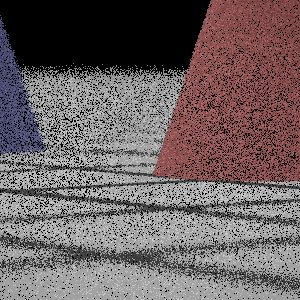My Implementation (Sampling 2)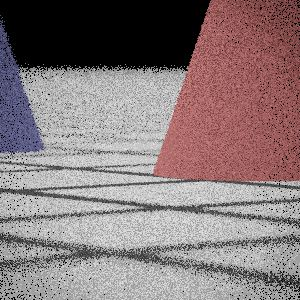My Implementation (Sampling 3)

#### Wide-Angle Lens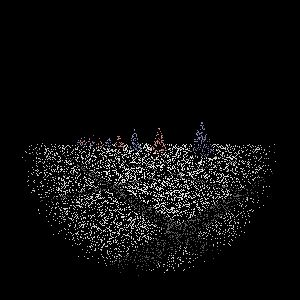My Implementation (Sampling 1)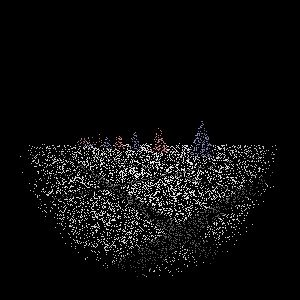My Implementation (Sampling 2)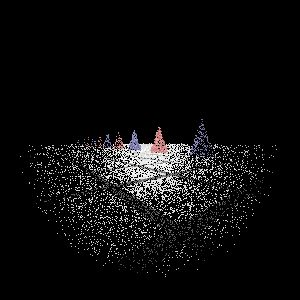My Implementation (Sampling 3)

## Final Images Rendered Compare 512 samples

#### Gauss Lens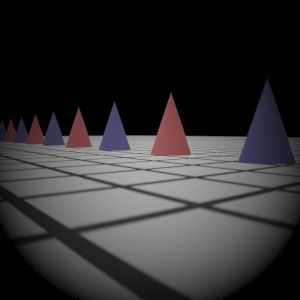Reference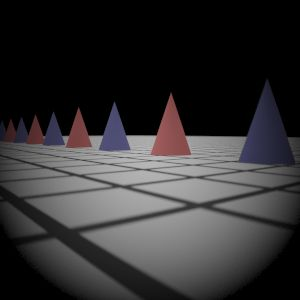My Implementation (Sampling 1)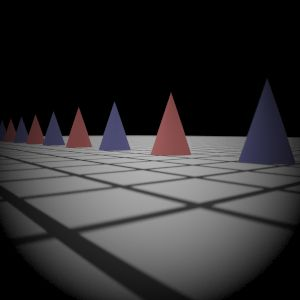My Implementation (Sampling 2)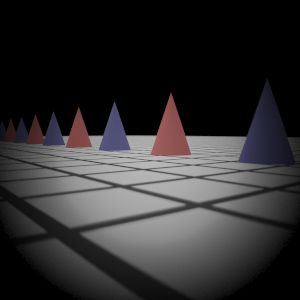My Implementation (Sampling 3)

#### Fisheye Lens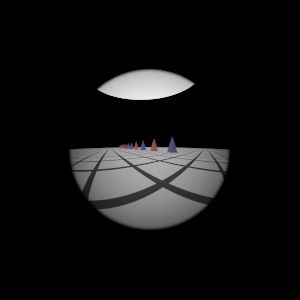Reference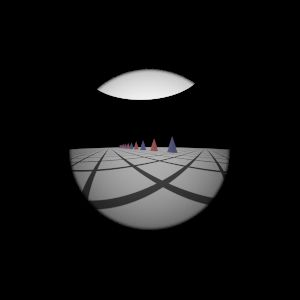My Implementation (Sampling 1)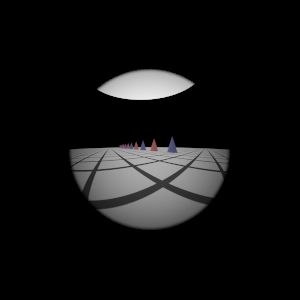My Implementation (Sampling 2)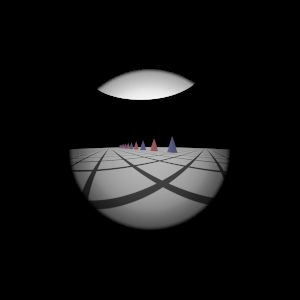My Implementation (Sampling 3)

#### Telephoto Lens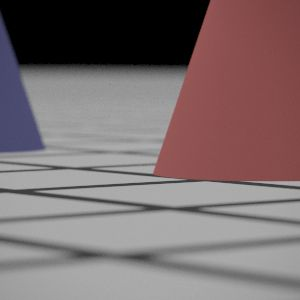Reference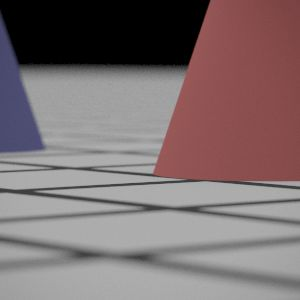My Implementation (Sampling 1)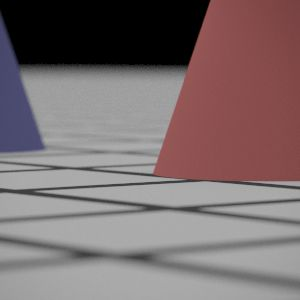My Implementation (Sampling 2)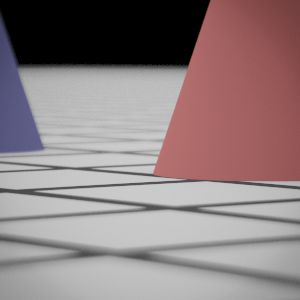My Implementation (Sampling 3)

#### Wide-Angle Lens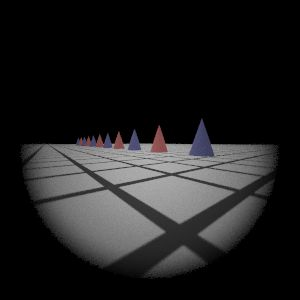Reference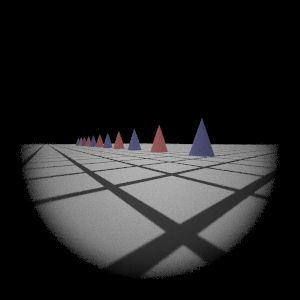My Implementation (Sampling 1)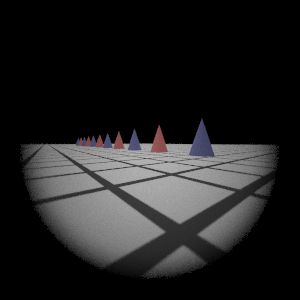My Implementation (Sampling 2)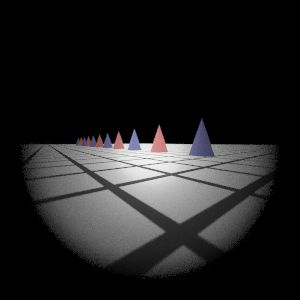My Implementation (Sampling 3)
Read More +

# pbrt-v2 加速結構 Height Field 改寫

### 目標工作

Height Field 是一個高度場，測資中以山脈構造為主，等間隔取樣每一平面上的每一點高度的場景，當間隔越小越接近真實場景。原生寫法是將點放置在三維空間，轉換成一堆三角網格，將其丟入 KD-tree 或者是 BVH 等加速結構，加快光線模擬找交點 (三維空間中，一射線和一多面體的交點) 的效能。而現在挑戰用 3D-DDA 的方式進行交點查找。

1. 預先將預處理每個 3D Triangle 座標 (消耗空間)
2. 需要時，再創建 Triangle 出來測試 (消耗時間)

#### Phong 圓滑化

Phong interpolation 方法在三角形上面操作時，需要得知三個頂點的法向量，給予 height field 的資訊，並不曉得每一個頂點的法向量為何。得到每一個頂點的法向量，採用預先處理所有頂點的法向量並儲存起來，因為不斷地正規化是很耗時間的 (float sqrt(float x) 儘管採用 fast inverse square root 算法，也要極力避免多餘的運算)。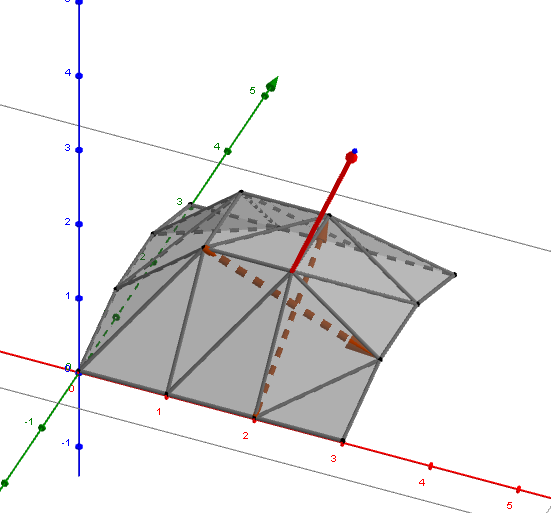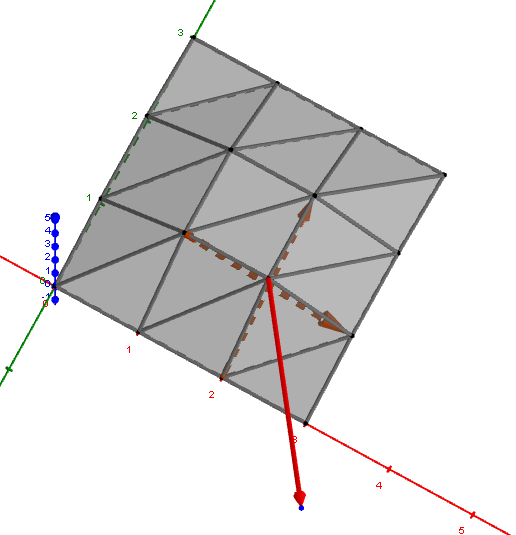##### Case 1 邊緣
$$N_x = \begin{bmatrix} 0 & 0 & 0 \\ -1 & 1 & 0 \\ 0 & 0 & 0 \\ \end{bmatrix}, \; N_y = \begin{bmatrix} 0 & 0 & 0 \\ 0 & 1 & 0 \\ 0 & -1 & 0 \\ \end{bmatrix}$$
##### Case 2 非邊緣
$$N_x = \begin{bmatrix} 0 & 0 & 0 \\ -1 & 0 & 1 \\ 0 & 0 & 0 \\ \end{bmatrix}, \; N_y = \begin{bmatrix} 0 & 1 & 0 \\ 0 & 0 & 0 \\ 0 & -1 & 0 \\ \end{bmatrix}$$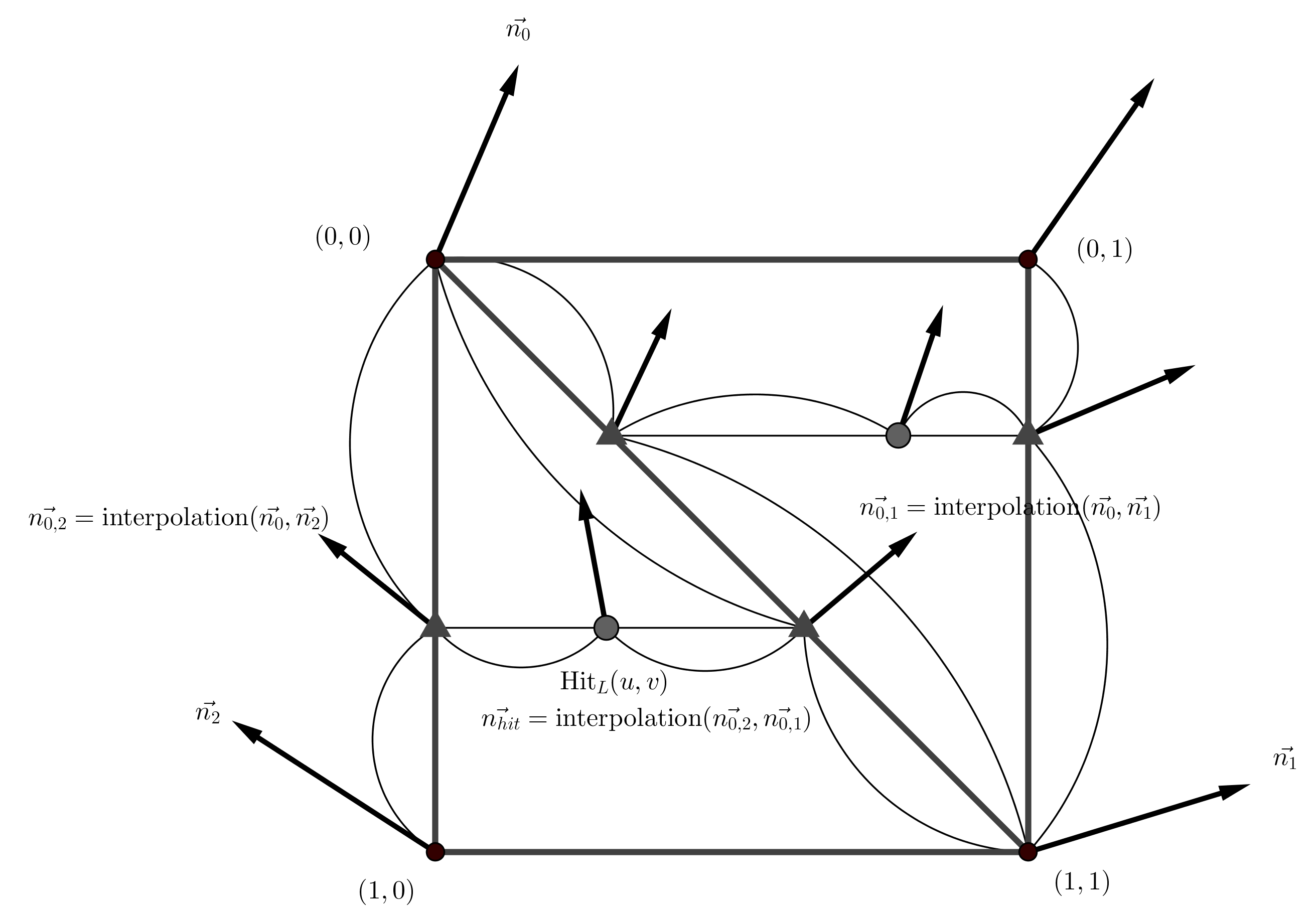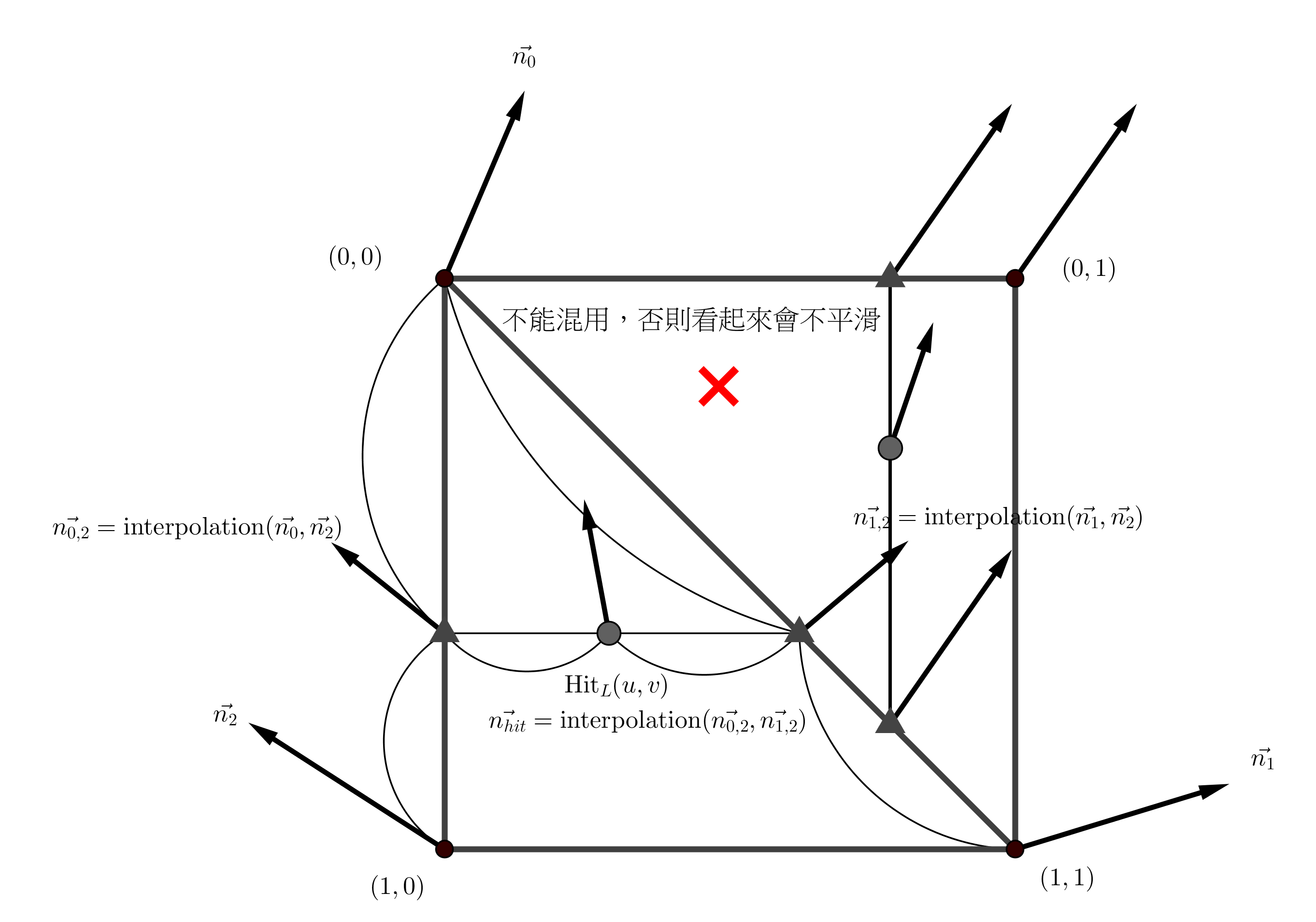### Final Images Rendered with my implementation of heightfield.cpp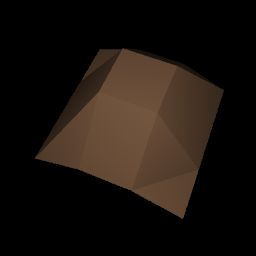hftest.pbrt (without Phong interpolation) Timings: Original: 0.125 seconds My implementation: 0.130 seconds (104% original)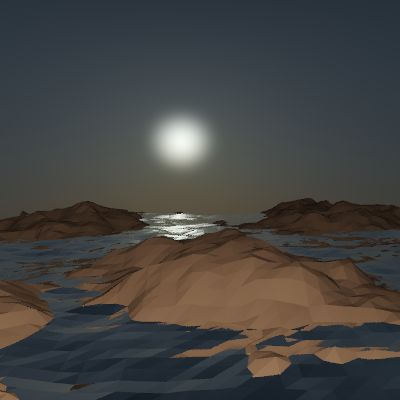landsea-0.pbrt (without Phong interpolation) Timings: Original: 0.815 seconds My implementation: 1.050 seconds (128% original)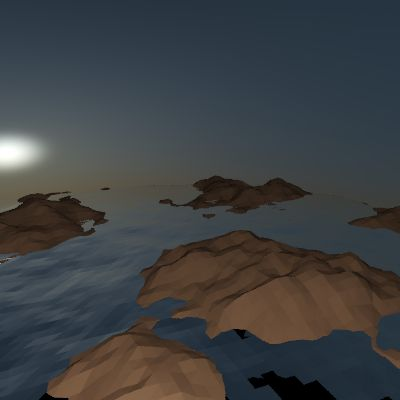landsea-1.pbrt (without Phong interpolation) Timings: Original: 0.850 seconds My implementation: 1.020 seconds (120% original)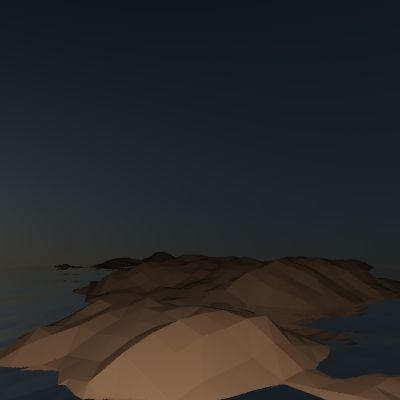landsea-2.pbrt (without Phong interpolation) Timings: Original: 0.780 seconds My implementation: 0.870 seconds (115% original)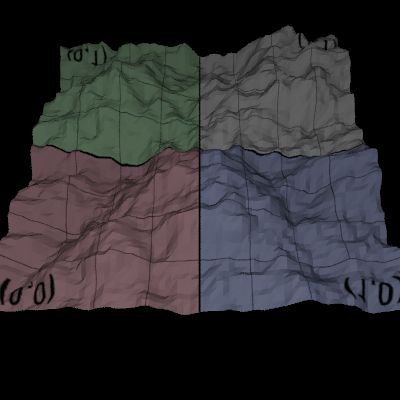texture.pbrt (without Phong interpolation) Timings: Original: 0.450 seconds My implementation: 0.520 seconds (120% original)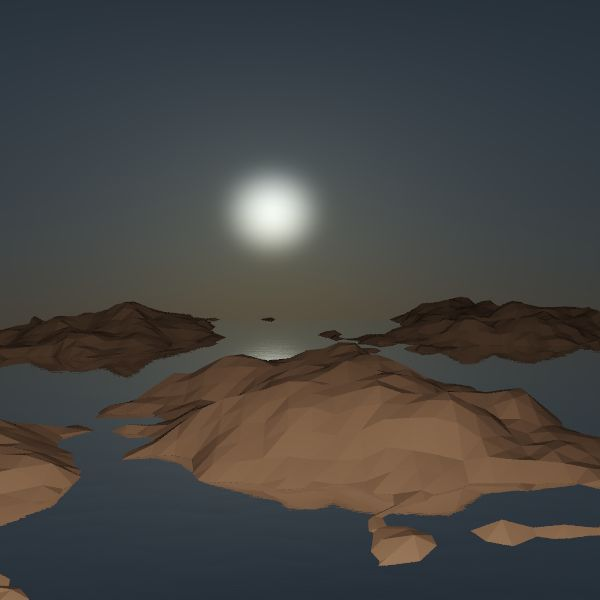landsea-big.pbrt (without Phong interpolation) Timings: Original: 6.200 seconds My implementation: 2.200 seconds (35% original)

### Final Images Rendered with my implementation of heightfield.cpp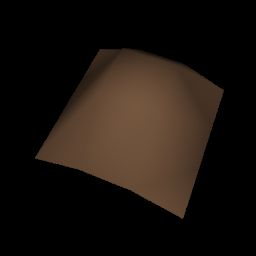hftest.pbrt (with Phong interpolation) Timings: My implementation: 0.135 seconds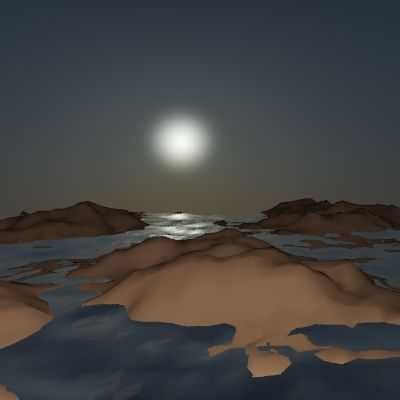landsea-0.pbrt (with Phong interpolation) Timings: My implementation: 1.100 seconds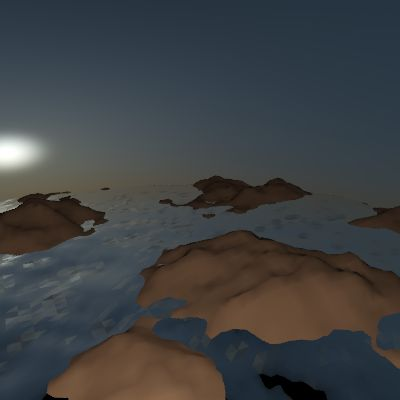landsea-1.pbrt (with Phong interpolation) Timings: My implementation: 1.050 seconds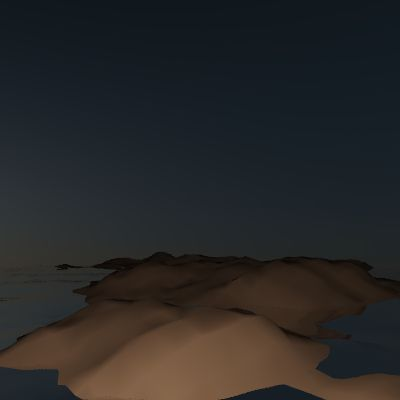landsea-2.pbrt (with Phong interpolation) Timings: My implementation: 0.900 seconds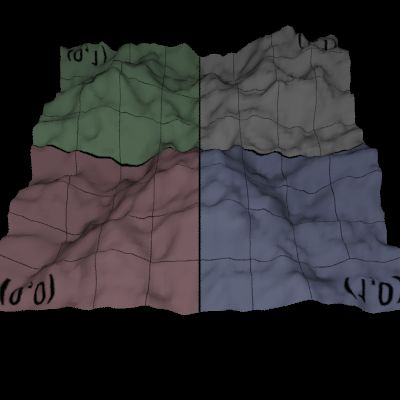texture.pbrt (with Phong interpolation) Timings: My implementation: 0.530 seconds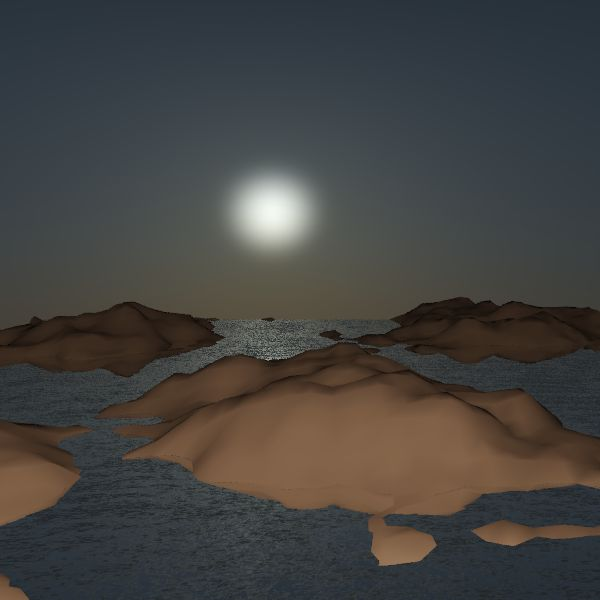landsea-big.pbrt (with Phong interpolation) Timings: My implementation: 2.300 seconds
Read More +